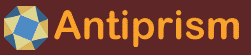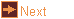# symmetro - polyhedra with regular polygons at symmetry axes

 Usage    |    Examples    |    Notes

## Usage

```

Usage: symmetro [options]

Symmetrohedra and Twisters are created by placing equilateral polygons centered
on the symmetry axes of Icosahedral, Octahedral, Tetrahedral, or Dihedral
symmetry. The sides of the polygons will be a multiple number of the axis
reflection number. Axes are numbered as 0, 1 and 2

It is possible to generate models such that the polygons intersect. If a
collision is detected, convex hull will be suppressed

options -k, -t, -s and -c cannot be used together but one needs to be specified

Options
-h,--help this help message (run 'off_util -H help' for general help)
--version version information
-v        verbose output
-l <lim>  minimum distance for unique vertex locations as negative exponent
(default: 12 giving 1e-12)
-o <file> write output to file (default: write to standard output)

Program Options
-k <s,l,m,n,a> Kaplan-Hart notation. Generate Symmetrohedra based on a study
by Craig S. Kaplan and George W. Hart (http://www.georgehart.com).
Url: https://cs.uwaterloo.ca/~csk/Old/projects/symmetrohedra/
Pdf: https://antiprism.com/misc/kaplan_hart_bridges2001.pdf
s: symmetry type of Symmetrohedra. sets {p,q,2}
I-icosahedral {5,3,2} O-octahedral {4,3,2} T-tetrahedral {3,3,2}
l,m,n: multipliers for axis polygons. Separated by commas, two
multipliers may be * or 0, no more than two positive integers
a: face rotation type: vertex=1, edge=0  (default: 1)
example: -k i,2,*,4,e
-t <s[p,q]i,m1,m2> Twister notation. Generate twister models.
s: symmetry. I-icosahedral, O-octahedral, T-tetrahedral, D-dihedral
p,q: rotational order of each of the two axes. may be swapped
i: (default: 1): integer to select between non-equivalent pairs of
axes having the same symmetry group and rotational orders
m1,m2: an integer multiplier for each axis. i.e. m1*p and m2*q
also can be entered as m1/d, m2/d fractional values
e.g. T[3,2],1,2  I[5,2]2,1/2,3  D7[7,3],1,2  D11[2,2]5,2,2
Axis pairs are from the following
T: [3,3], [3,2], [2,2]
O: [4,4], [4,3], [4,2]x2, [3,3], [3,2]x2, [2,2]x2
I: [5,5], [5,3]x2, [5,2]x3, [3,3]x2, [3,2]x4, [2,2]x4
Dn:[n,2], [2,2]x(n/2 rounded down)
-s <n/d:D>,s S symmetry twisters. denominator d optional (default: 1)
optional D substitutes a polygon of n/D in place of n/d
optional s: symmetry override c - C symmetry
-c <n1/d1:D1,n2/d2:D2,s,v> C symmetry twisters
optional d denominator (default: 1)
optional D substitutes a polygon of n/D in place of n/d
optional n2 can differ from n1. if not specified n2=n1, d2=d1
optional s: symmetry: c - C (default), h - Ch, v - Cv, d - D
optional v: vertex index of radial polygon to bring to z plane
v of -1 is original position (default: index of largest z)
-M <opt>  mirroring (may create compound). Can be x, y or z (default: none)
-a <a,n>  a in degrees of rotation given to polygon applied to optional
axis n (default: 0). A suffix of 'e' may be used, where 1.0e is
half the central angle of an edge. Then a is a multiplier. For
example 2e. A suffix of x is like e but with a half turn offset
-r <r,n>  set the edge length of the polygon on axis n (default: 0)
to r. Must be non-negative. The default edge length is 1
-A <a>    a in degrees is angle between axes (default: calculated)

Scene Options
-C <mode> convex hull. polygons=1, suppress=2, force=3, auto=4 (default: 4)
-q <args> include frame elements in output
r - rhombic tiling edges, a - rotation axes (default: none)
-O <dist> amount to offset the first polygon to avoid coplanarity with the
second polygon, for example 0.0001 (default: 0.0)
-x        remove any free faces that are produced

Coloring Options (run 'off_util -H color' for help on color formats)
-V <col>  vertex color (default: gold)
-E <col>  edge color   (default: lightgray)
-f <col>  face color. Or use method for using color in map (default: a)
keyword: none - sets no color
a - color by axis number
n - color by number of sides
-T <tran> face transparency. valid range from 0 (invisible) to 255 (opaque)
-D        don't cover digons with edge color
-Q <col>  frame color  (default: skyblue3)
-m <maps> color maps for faces to be tried in turn (default: m1)
keyword m1: red,blue,yellow,darkgreen
note: position 4 color is for faces added by convex hull
keyword m2: approximating colors in the symmetrohedra pdf file
keyword m3: red,darkorange1,yellow,darkgreen,cyan,blue,magenta,
white,gray50,black (default when -f n)
(built in maps start at index 3 when -f n)

```

## Examples

Make a Cuboctahedron (U7)

```symmetro -k o,1,1,0,e | antiview
symmetro -t O[3,3],1,1 | antiview
```

Like a Rhombicuboctahedron, but scale the squares on the 4-fold axes by 2.
```symmetro -k o,1,1,0 -r 2  | antiview
symmetro -t O[4,3],1,1 -r 2 | antiview
```

Make an approximate Snub Cube (U12)
```symmetro -k o,1,0,1 -a 28.53243959961367 | antiview
symmetro -t O[4,2],1,1 -a 28.53243959961367 -C f | antiview
```

Make a 'twister' by suppressing the convex hull and varying the twist parameter
```symmetro -k t,1,1,0 -C s -a 15 | antiview
symmetro -t T[3,3],1,1 -C s -a 15 | antiview
```

Make an Small Stellated Dodecahedron (U34)
```symmetro -s 5:2 -a 2e | antiview -v 0.03
```

Make an Icosidodecahedron (U24)
```symmetro -c 5,5,d | antiview
```

Make an Pentagonal Orthobirotunda (Johnson Solid J34)
```symmetro -c 5,5,h | antiview
```

Bowtie models from Kaplan-Hart paper Figure 3 in order of appearance
```symmetro -k t,2,3,*,e | antiview
symmetro -k o,2,3,*,e | antiview
symmetro -k o,3,2,*,e | antiview
symmetro -k i,2,3,*,e | antiview
symmetro -k i,3,2,*,e | antiview
symmetro -k t,1,2,*,v | antiview
symmetro -k o,1,2,*,v | antiview
symmetro -k o,2,1,*,v | antiview
symmetro -k i,1,2,*,v | antiview
symmetro -k i,2,1,*,v | antiview
```

Jamnitzer models from Kaplan-Hart paper Figure 7 in order of appearance
```symmetro -k o,2,2,0,v -r 1.06680419358835388 -f n -m m2 | antiview
symmetro -k o,3,3,0,v -r 1.04966777114351 -f n -m m2 | antiview -v 0.03
symmetro -k i,2,2,0,v -r 1.09818547139510914 -f n -m m2 | antiview -v 0.03
symmetro -k i,3,3,0,v -r 1.0726110096817 -f n -m m2 | antiview -v 0.05
```

LCM models from Kaplan-Hart paper Figure 8 in order of appearance
```symmetro -k t,2,0,3,e -f n -m m2 | antiview
symmetro -k t,2,0,3,v -f n -m m2 | antiview
symmetro -k o,1,0,2,v -f n -m m2 | antiview
symmetro -k o,3,4,*,e -f n -m m2 | antiview
symmetro -k o,3,4,*,v -f n -m m2 | antiview
symmetro -k o,*,2,3,e -f n -m m2 | antiview
symmetro -k o,*,2,3,v -f n -m m2 | antiview
symmetro -k i,3,5,*,e -f n -m m2 | antiview
symmetro -k i,3,5,*,v -f n -m m2 | antiview
symmetro -k i,2,*,5,e -f n -m m2 | antiview
symmetro -k i,2,*,5,v -f n -m m2 | antiview
symmetro -k i,*,2,3,e -f n -m m2 | antiview
symmetro -k i,*,2,3,v -f n -m m2 | antiview
```

Near miss models from Kaplan-Hart paper Figure 9 in order of appearance
```symmetro -k o,*,3,*,e -f n -m m2 | antiview
symmetro -k i,1,2,*,v -f n -m m2 -r 1.07046626932,1 | antiview
symmetro -k i,2,*,3,e -f n -m m2 | antiview
```

Appealing models from Kaplan-Hart paper Figure 10 in order of appearance
```symmetro -k i,2,2,*,1 -f n -m m2 -r 2.03614784182,1 | antiview -v 0.03
symmetro -k i,2,1,*,1 -f n -m m2 -r 1.9021130325885 | antiview -v 0.03
symmetro -k i,2,2,*,1 -f n -m m2 | antiview -v 0.05
symmetro -k i,4,3,*,e -f n -m m2 | antiview -v 0.07
symmetro -k i,2,3,*,1 -f n -m m2 | antiview -v 0.05
symmetro -k i,2,3,*,1 -f n -m m2 -r 1.583841856265,1 | antiview -v 0.05
symmetro -k i,2,4,*,e -f n -m m2 | antiview -v 0.07
symmetro -k i,2,4,*,1 -f n -m m2 -r 1.05398768021455,1 | antiview -v 0.07
symmetro -k i,3,1,*,1 -f n -m m2 | antiview -v 0.07
symmetro -k i,3,2,*,1 -f n -m m2 | antiview -v 0.07
symmetro -k i,*,2,2,1 -f n -m m2 | antiview -v 0.07
symmetro -k i,*,1,4,e -f n -m m2 -C 3 | antiview -v 0.06
symmetro -k i,3,4,*,v -f n -m m2 -r 1.5665309813,1 -l 8 | antiview -v 0.03
symmetro -k i,3,4,*,e -f n -m m2 | antiview -v 0.06
symmetro -k i,3,5,0,v -f n -m m2 -r 1.3159206178527,1 | antiview -v 0.03
symmetro -k i,4,2,*,e -f n -m m2 | antiview -v 0.06
symmetro -k i,*,1,4,1 -f n -m m2 -C 3 | antiview -v 0.06
symmetro -k i,1,*,2,1 -f n -m m2 | antiview -v 0.06
symmetro -k i,1,*,3,e -f n -m m2 | antiview -v 0.06
symmetro -k i,2,*,3,1 -f n -m m2 | antiview -v 0.06
```

## Notes

symmetro was written by Roger Kaufman.